# Merge Sort Algorithm | Example | Time Complexity

## Merge Sort-

• Merge sort is a famous sorting algorithm.
• It uses a divide and conquer paradigm for sorting.
• It divides the problem into sub problems and solves them individually.
• It then combines the results of sub problems to get the solution of the original problem.

## How Merge Sort Works?

Before learning how merge sort works, let us learn about the merge procedure of merge sort algorithm.

The merge procedure of merge sort algorithm is used to merge two sorted arrays into a third array in sorted order.

Consider we want to merge the following two sorted sub arrays into a third array in sorted order-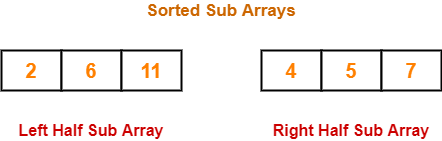The merge procedure of merge sort algorithm is given below-

```// L : Left Sub Array , R : Right Sub Array , A : Array
merge(L, R, A)
{
nL = length(L)    // Size of Left Sub Array
nR = length(R)    // Size of Right Sub Array
i = j = k = 0
while(i<nL && j<nR)
{
/* When both i and j are valid i.e. when both the sub arrays have elements to insert in A */
if(L[i] <= R[j])
{
A[k] = L[i]
k = k+1
i = i+1
}
else
{
A[k] = R[j]
k = k+1
j = j+1
}
}
// Adding Remaining elements from left sub array to array A
while(i<nL)
{
A[k] = L[i]
i = i+1
k = k+1
}
// Adding Remaining elements from right sub array to array A
while(j<nR)
{
A[k] = R[j]
j = j+1
k = k+1
}
}
```

The above merge procedure of merge sort algorithm is explained in the following steps-

### Step-01:

• Create two variables i and j for left and right sub arrays.
• Create variable k for sorted output array.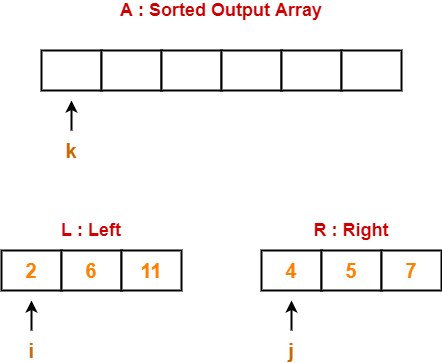### Step-02:

• We have i = 0, j = 0, k = 0.
• Since L < R, so we perform A = L i.e. we copy the first element from left sub array to our sorted output array.
• Then, we increment i and k by 1.

Then, we have-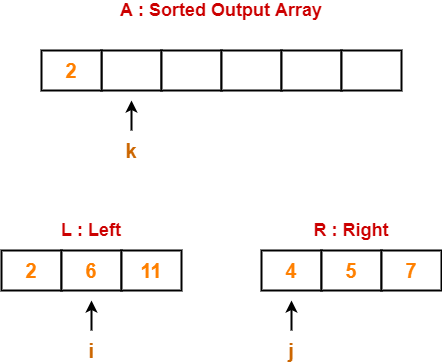### Step-03:

• We have i = 1, j = 0, k = 1.
• Since L > R, so we perform A = R i.e. we copy the first element from right sub array to our sorted output array.
• Then, we increment j and k by 1.

Then, we have-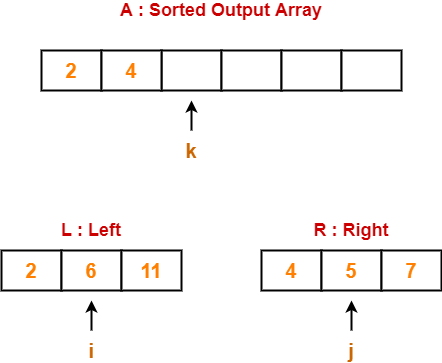### Step-04:

• We have i = 1, j = 1, k = 2.
• Since L > R, so we perform A = R.
• Then, we increment j and k by 1.

Then, we have-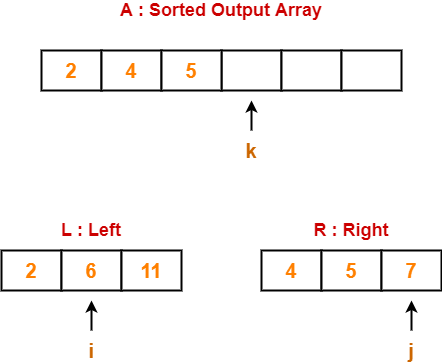### Step-05:

• We have i = 1, j = 2, k = 3.
• Since L < R, so we perform A = L.
• Then, we increment i and k by 1.

Then, we have-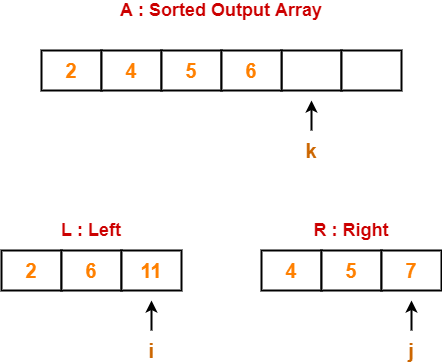### Step-06:

• We have i = 2, j = 2, k = 4.
• Since L > R, so we perform A = R.
• Then, we increment j and k by 1.

Then, we have-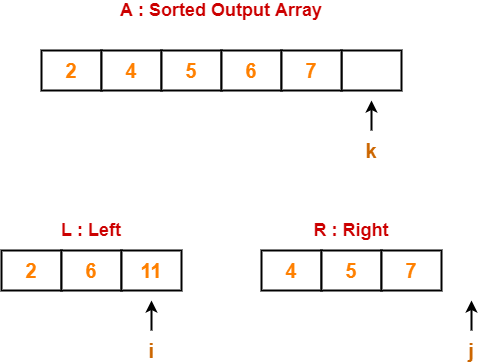### Step-07:

• Clearly, all the elements from right sub array have been added to the sorted output array.
• So, we exit the first while loop with the condition while(i<nL && j<nR) since now j>nR.
• Then, we add remaining elements from the left sub array to the sorted output array using next while loop.

Finally, our sorted output array is-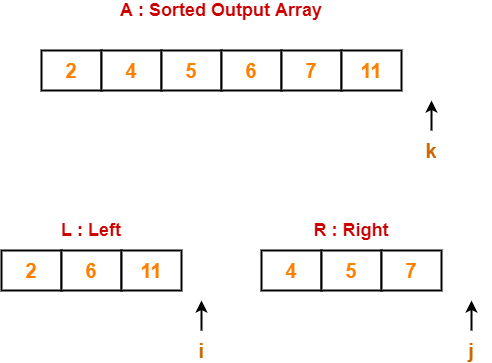Basically,

• After finishing elements from any of the sub arrays, we can add the remaining elements from the other sub array to our sorted output array as it is.
• This is because left and right sub arrays are already sorted.

### Time Complexity

The above mentioned merge procedure takes Θ(n) time.

This is because we are just filling an array of size n from left & right sub arrays by incrementing i and j at most Θ(n) times.

## Merge Sort Algorithm-

Merge Sort Algorithm works in the following steps-

• It divides the given unsorted array into two halves- left and right sub arrays.
• The sub arrays are divided recursively.
• This division continues until the size of each sub array becomes 1.
• After each sub array contains only a single element, each sub array is sorted trivially.
• Then, the above discussed merge procedure is called.
• The merge procedure combines these trivially sorted arrays to produce a final sorted array.

The division procedure of merge sort algorithm which uses recursion is given below-

```// A : Array that needs to be sorted
MergeSort(A)
{
n = length(A)
if n<2 return
mid = n/2
left = new_array_of_size(mid)       // Creating temporary array for left
right = new_array_of_size(n-mid)    // and right sub arrays
for(int i=0 ; i<=mid-1 ; ++i)
{
left[i] = A[i]                  // Copying elements from A to left
}
for(int i=mid ; i<=n-1 ; ++i)
{
right[i-mid] = A[i]             // Copying elements from A to right
}
MergeSort(left)                    // Recursively solving for left sub array
MergeSort(right)                   // Recursively solving for right sub array
merge(left, right, A)              // Merging two sorted left/right sub array to final array
}```

## Merge Sort Example-

Consider the following elements have to be sorted in ascending order-

6, 2, 11, 7, 5, 4

The merge sort algorithm works as-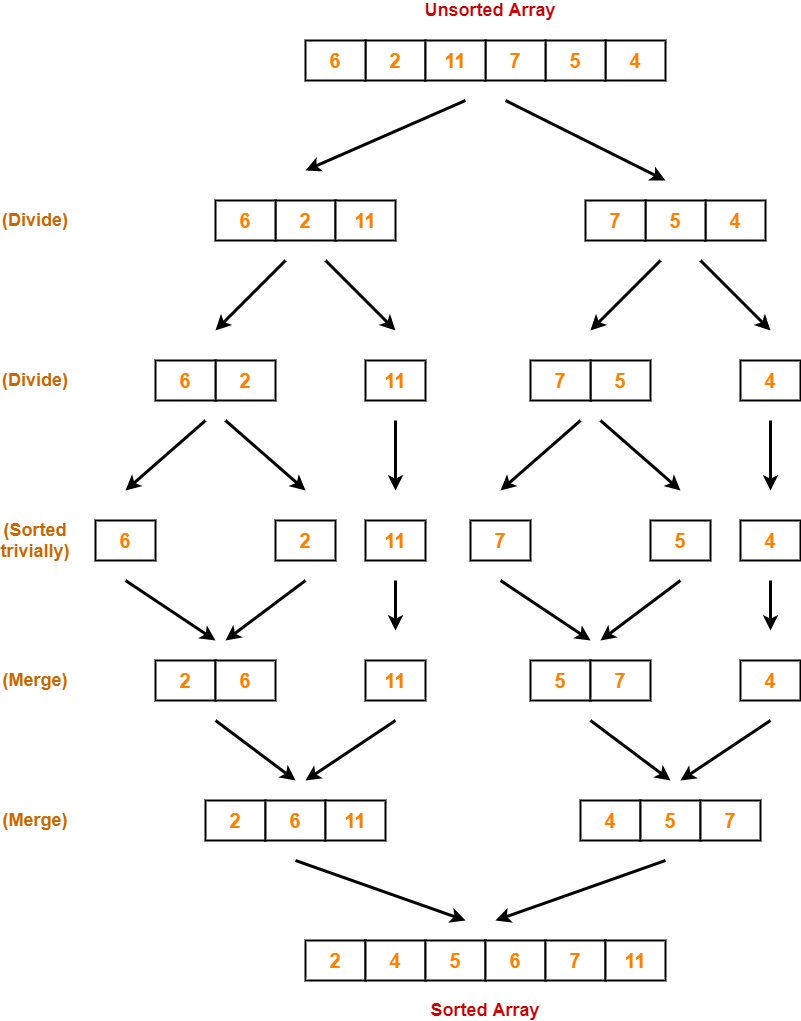## Time Complexity Analysis-

In merge sort, we divide the array into two (nearly) equal halves and solve them recursively using merge sort only.

So, we have-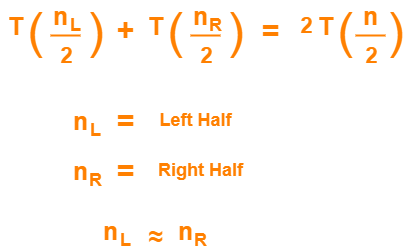Finally, we merge these two sub arrays using merge procedure which takes Θ(n) time as explained above.

If T(n) is the time required by merge sort for sorting an array of size n, then the recurrence relation for time complexity of merge sort is-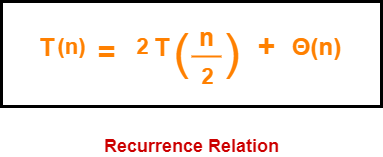On solving this recurrence relation, we get T(n) = Θ(nlogn).

Thus, time complexity of merge sort algorithm is T(n) = Θ(nlogn).

## Space Complexity Analysis-

• Merge sort uses additional memory for left and right sub arrays.
• Hence, total Θ(n) extra memory is needed.

## Properties-

Some of the important properties of merge sort algorithm are-

• Merge sort uses a divide and conquer paradigm for sorting.
• Merge sort is a recursive sorting algorithm.
• Merge sort is a stable sorting algorithm.
• Merge sort is not an in-place sorting algorithm.
• The time complexity of merge sort algorithm is Θ(nlogn).
• The space complexity of merge sort algorithm is Θ(n).

### NOTE

Merge sort is the best sorting algorithm in terms of time complexity Θ(nlogn)

if we are not concerned with auxiliary space used.

## Problem-

Assume that a merge sort algorithm in the worst case takes 30 seconds for an input of size 64. Which of the following most closely approximates the maximum input size of a problem that can be solved in 6 minutes? (GATE 2015)

1. 256
2. 512
3. 1024
4. 2048

## Solution-

We know, time complexity of merge sort algorithm is Θ(nlogn).

### Step-01:

It is given that a merge sort algorithm in the worst case takes 30 seconds for an input of size 64.

So, we have-

k x nlogn = 30 (for n = 64)

k x 64 log64 = 30

k x 64 x 6 = 30

From here, k = 5 / 64.

### Step-02:

Let n be the maximum input size of a problem that can be solved in 6 minutes (or 360 seconds).

Then, we have-

k x nlogn = 360

(5/64) x nlogn = 360 { Using Result of Step-01 }

nlogn = 72 x 64

nlogn = 4608

On solving this equation, we get n = 512.

Thus, correct option is (B).

To gain better understanding about Merge Sort Algorithm,

Watch this Video Lecture

Next Article- Quick Sort

### Other Popular Sorting Algorithms-

Get more notes and other study material of Design and Analysis of Algorithms.

Watch video lectures by visiting our YouTube channel LearnVidFun.

SummaryArticle Name
Merge Sort Algorithm | Example | Time Complexity
Description
Merge Sort is a famous sorting algorithm that uses divide and conquer paradigm. Merge Sort Algorithm with Example is given. The time complexity of Merge Sort Algorithm is Θ(nlogn) and its space complexity is Θ(n).
Author
Publisher Name
Gate Vidyalay
Publisher Logo